## Example Questions

### Example Question #2 : How To Find The Length Of The Diagonal Of A Rectangle

What is the length of the diagonal of a rectangle that is 3 feet long and 4 feet wide?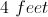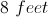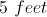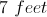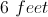Explanation:

The diagonal of the rectangle is equivalent to finding the length of the hypotenuse of a right triangle with sides 3 and 4. Using the Pythagorean Theorem: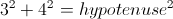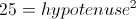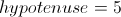Therefore the diagonal of the rectangle is 5 feet.

### Example Question #3 : How To Find The Length Of The Diagonal Of A Rectangle

The length and width of a rectangle are in the ratio of 3:4. If the rectangle has an area of 108 square centimeters, what is the length of the diagonal?

15 centimeters

18 centimeters

12 centimeters

24 centimeters

9 centimeters

15 centimeters

Explanation:

The length and width of the rectangle are in a ratio of 3:4, so the sides can be written as 3x and 4x.

We also know the area, so we write an equation and solve for x:

(3x)(4x) = 12x= 108.

x2 = 9

x = 3

Now we can recalculate the length and the width:

length = 3x = 3(3) = 9 centimeters

width = 4x = 4(3) = 12 centimeters

Using the Pythagorean Theorem we can find the diagonal, c:

length2 + width2 = c2

92 + 12= c2

81 + 144 = c2

225 = c2

= 15 centimeters

### Example Question #1 : How To Find The Length Of The Diagonal Of A Rectangle

Find the length of the diagonal of a rectangle whose sides are 8 and 15.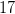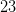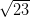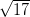Explanation:

To solve. simply use the Pythagorean Theorem where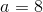and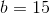Thus,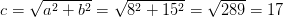### Example Question #2 : How To Find The Length Of The Diagonal Of A Rectangle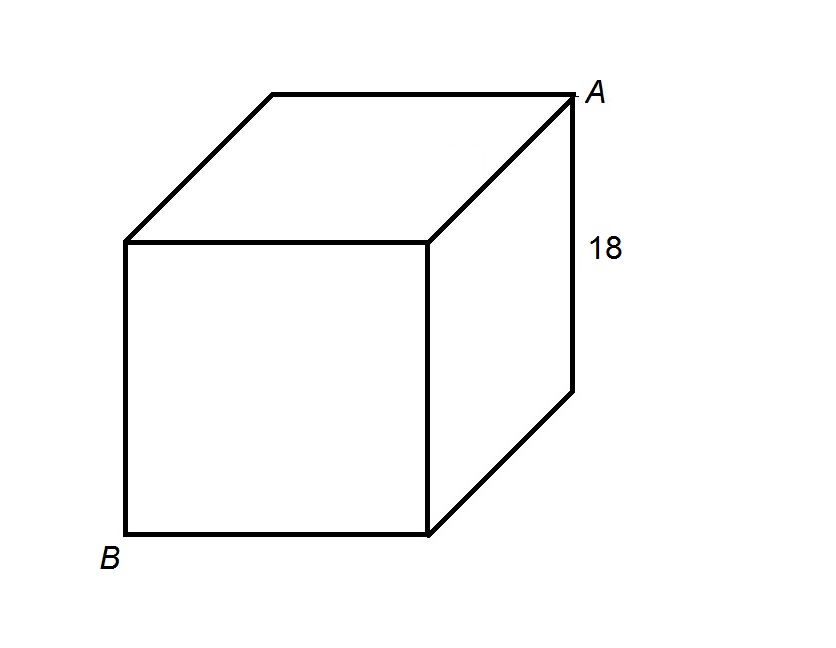The above figure depicts a cube, each edge of which has length 18. Give the length of the shortest path from Point A to Point B that lies completely along the surface of the cube.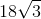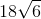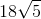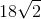Explanation:

The shortest path is along two of the surfaces of the prism. There are three possible choices - top and front, right and front, and rear and bottom - but as it turns out, since all faces are (congruent) squares, all three paths have the same length. One such path is shown below, with the relevant faces folded out: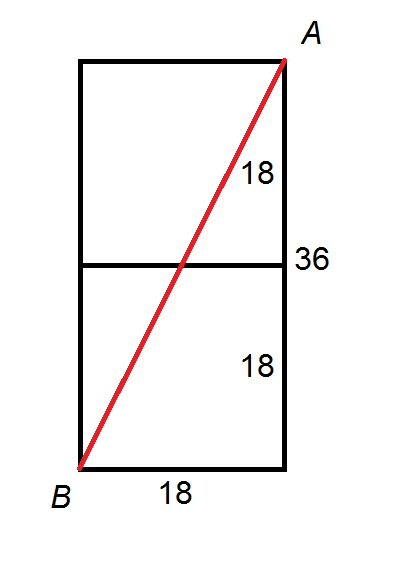The length of the path can be seen to be equal to that of the diagonal of a rectangle with length and width 18 and 36, so its length can be found by applying the Pythagorean Theorem. Substituting 18 and 36 forand: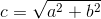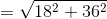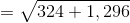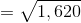Applying the Product of Radicals Rule: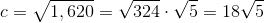.